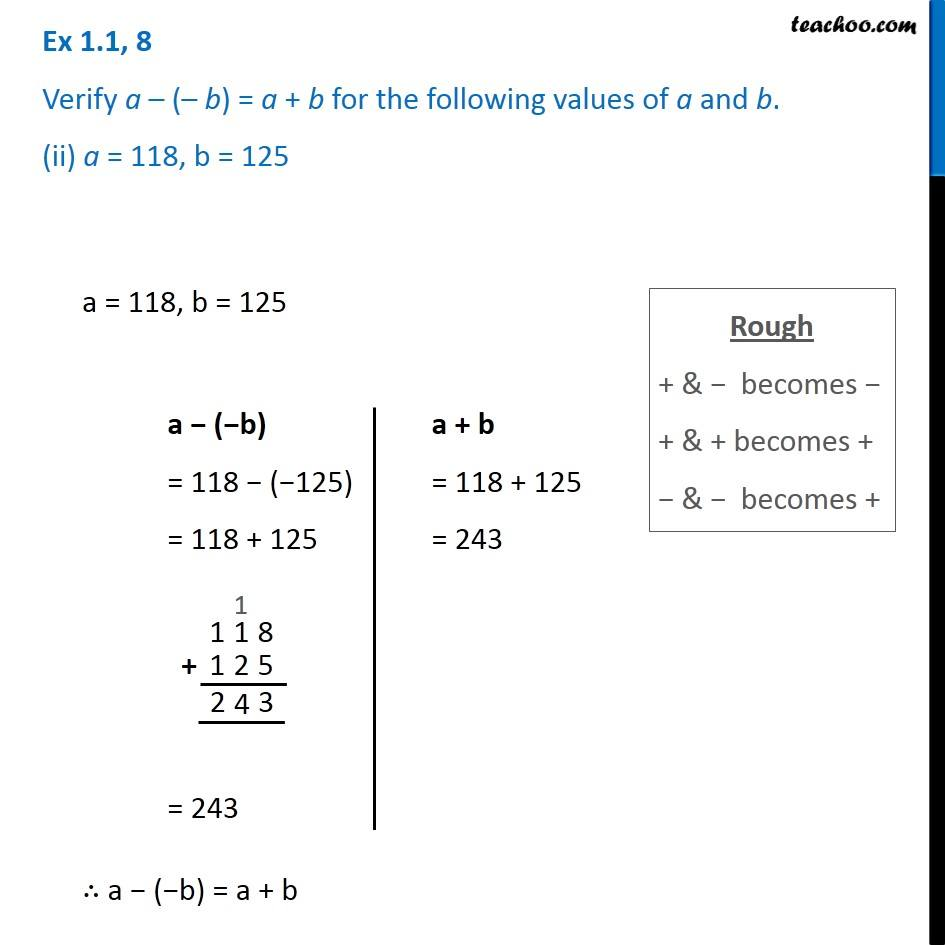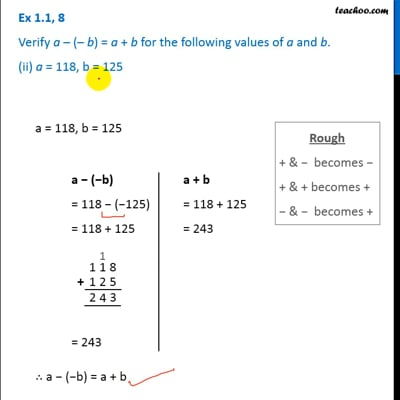Ex 1.1

Chapter 1 Class 7 Integers
Serial order wiseThis video is only available for Teachoo black users

Get live Maths 1-on-1 Classs - Class 6 to 12

### Transcript

Ex 1.1, 8 Verify a – (– b) = a + b for the following values of a and b. (ii) a = 118, b = 125 Rough + & − becomes − + & + becomes + − & − becomes + a = 118, b = 125 a − (−b) = 118 − (−125) = 118 + 125 = 243 a + b = 118 + 125 = 243 ∴ a − (−b) = a + b